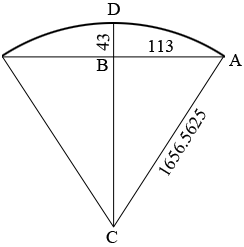SEARCH HOMEMath Central Quandaries & QueriesQuestion from Gerry: Looking to make a full size template for an arched window. Need increments every 16". The radius is 138' 0 9/16" , the chord is 226" and the rise at center of chord is 43"Hi Gerry,

I think there is something wrong with your question. First I assumed the arc at the top of the window is an arc of a circle. Since $138 \times 12 = 1656$ and $\large \frac{9}{16} = 0.5625$ the radius of the circle is 1656.5625 inches. Here is my diagram of what you described, All the dimensions are in inches. My diagram is not to scale.Since the triangle $ABC$ is a right triangle I can use Pythagoras' Theorem to conclude that

$|BC|^2 = |CA|^2 - |AB|^2 = 1656.5625^2 - 113^2 = 2731430.3164$

so

$|BC| = 1652.7039 \mbox{ inches.}$

But this would mean that $|BD|,$ the rise at the center of the cord is $1656.5625 - 1652.7039 = 3.85$ inches rather than 43 inches.

I am not sure what is wrong but how do you know that the radius of the circle is 138' 0 9/16"?

PennyMath Central is supported by the University of Regina and The Pacific Institute for the Mathematical Sciences.